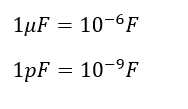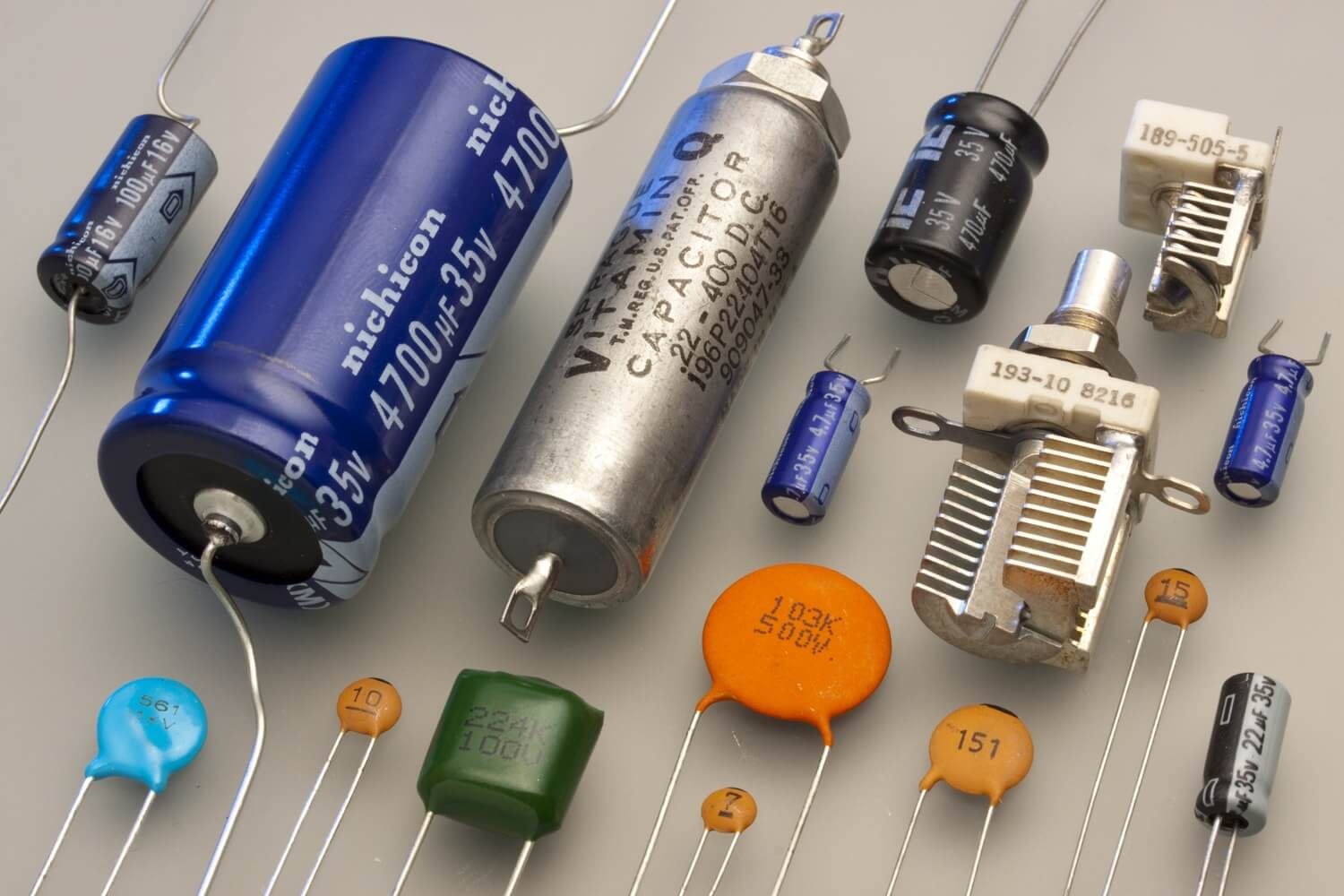# What is Capacitor | Types of Capacitor | What is Farad | Working Principle

## Capacitor:

Capacitor stores electric charge. It is looks like battery it stores energy in a different way. It is stores much energy in battery. It releases charge very faster. Capacitor is very useful that’s why it is used in all circuit boards.

It is one of the fundamental passive components. It is separately or jointly used with other circuit components such as inductor or resistor or others. But in AC Power circuit it is used in power factor correction. It is a two terminal device which stores energy in an electric field. It is consisting of two parallel plates.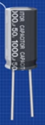They are made of conducting materials such as copper or silver or iron (mostly silver) and they are separated by a layer of dielectric material. The dielectric material is filled in between the capacitor’s terminal. Here, The dielectric material is an efficient insulator, so that it prevents electron flow across the terminal.

## History of Capacitor:

In 1745 the Leyden jar (former name of the capacitor) was competitively invented by Ewald Jurgen von Kleist – 4 Nov 1745. Pieter van Musschenbroek produced the first working example in Jan 1746, with the name coming from Leiden University. In 1747 William Watson discharges a Leyden jar through a circuit and comprehends electric current.

## Working Principle of Capacitor:

Positive Q+ as Plate A and Negative Q- as Plate B,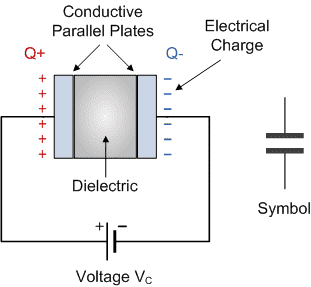Consider two parallel plates A and B, and A is connected with positive terminal of the voltage source and B is connected with a negative terminal of the same source. The electron flows from negative terminal and accumulates on the plate B developing negative charge, due to this the equal number of positive charges accumulate in plate A.

Here the electric field is established in the dielectric between the plates. The direction of electric field always drives electrons from the positively charged plate to positive terminal of the source. The amount of negative charge stored on plate B is equal to the amount of positive charge on the plate A. Due to this, the two plates A and B carry equal and opposite charges, since, there is a voltage across these two plates.

Let us consider voltage across the capacitor is Vc, and it is opposite that of applied voltage V. As the charge on the plate increases, the voltage across the plates also increases simultaneously. AT the same time, if the voltage across the parallel plates reaches to the source voltage V, then, there is not flow of electrons from the source.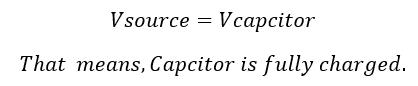The property of the capacitor is called as capacitance. The ability of a capacitor to store energy is measured by capacitance. It is defined as the ratio between the charge stored Q by a capacitor to the voltage V across the terminal. The capacitance is denoted as “C”.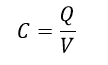Note: A capacitor is a device for temporarily storing electric charge.

In circuits capacitor acts a water tank and it stores energy. It releases and more interruption of supply. A capacitor is like a storage tank it can used for smooth out interruption to the supply.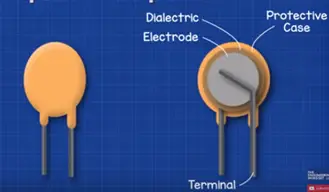One side of the capacitor is connected to the positive side of the circuit and other side is connected with negative side of the capacitor. The stripe in symbol indicates which side is negative. If we connect capacitor to battery the voltage will push the electron from negative terminal over the capacitor.

The electron will build up in the one plate of capacitor and other plate will turn and release some electrons. Electrons can pass through capacitor through capacitor because of insulating material eventually the capacitor is the same voltage as the battery. Then no more electrons will flow.

Learn More:   Motor Hp (Horse Power) Calculator DC, Single Phase & Three phase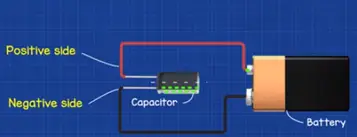There is a build up an electrons on one side this means we have stored energy. This can be released to do work because more electrons in one side to compare to other electron are negatively charged. This means we have one side negative and other side positive.

So there is difference in potential or voltage difference between the two. The voltages we are compare difference between two points. If we measure 1.5 V batteries then we read the difference between the 1.5V each end. But if we measure same end there voltage is zero there is no difference.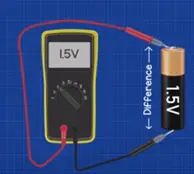If we measure the voltage difference between the two it build-up of electrons we still get a reading even when we disconnect the battery.

We remember a magnets opposite poles they attract each other when they are same poles they will oppose each other. Same build of negative charged electrons. They are attracted to positive charged particles of atom of the opposite plate.

They never reach each other because of the insulating material. This pole between the two sides electric field which holds electrons in place which other part is made.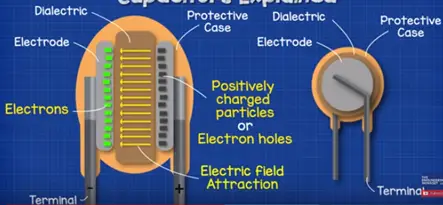If we place a small lamp in the circuit a power is exists electron will flow and reach the opposite side, so electrons flow through the electric lamp powering it and electrons will reach the opposite side of the capacitor.

Once we connect the battery again capacitor begin to charge this allows the interpret power supply and capacitor will provide power during this interruption.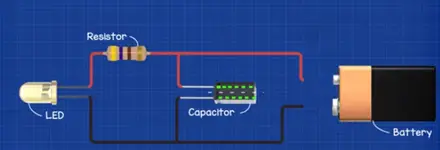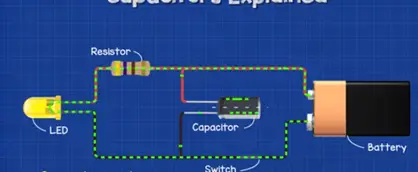### Where do we use capacitor?

They look little bit different but easy to spot in circuit boards. It represented in diagram.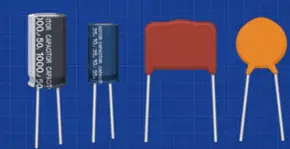They also represented in engineering drawings also.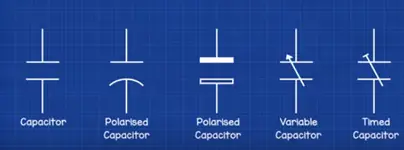The large capacitor is used in induction motors, fans and conditioner units. Even large one used in power factor for larger buildings.

On the side of the capacitor we can find two values that are capacitance and voltage.

Learn More:   7 Purpose of NGR Neutral Grounding Resistor Transformer & Generator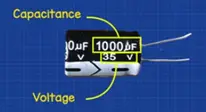### Symbol of the capacitor:

We can measure the capacitance of the capacitor in the unit of farads. This is mentioned as ‘F’ in capacitor. his is a very large unit.

In circuit boards typically use micro-farads. It is used like letter ‘μ’ other value is voltage which we can measure in volts ‘V’. In the capacitor the voltage is the maximum value which capacitor can handle. The capacitor is rated at certain voltage it exceeds the capacitor will explode.

Microfarad capacitors mainly used in electrical power system and power factor correction circuit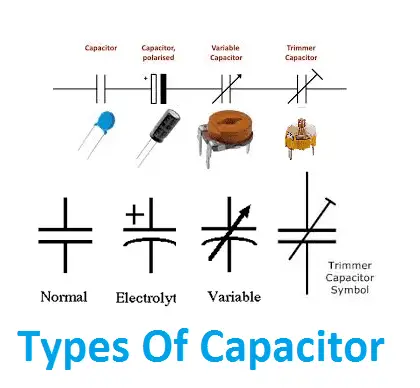The small capacitor in the range of below one microfarad Capacitors is mainly used in electronics circuit. There are many types of capacitors are in the market.